# 支持向量机在回归预测有机碳含量中的应用研究——以川东南地区为例Prediction of Organic Carbon Content Based on Rendezvous Graph and Support Vector Machine Regression—A Case Study of Maokou Formation in Southeast Sichuan Province

• 全文下载: PDF(2177KB)    PP.230-237   DOI: 10.12677/AG.2019.94026
• 下载量: 282  浏览量: 1,812   国家自然科学基金支持

四川盆地川东南地区为浅水–深水陆棚沉积环境，区内烃源岩发育，烃源岩富含大量有机质，最开始采用化学方法进行分析和判定，但是评价结果难以满足日益增长的生产需求，所以有机碳含量(TOC)计算模型作为一种有效的识别方式得到了广泛的应用。本文利用交会图法选出贡献率高的三条测井曲线：声波时差、自然伽马和深侧向电阻率，然后输入三条测井曲线值建立有机碳支持向量机回归预测模型。结果表明：有机碳含量在0.5以上时预测效果较好，当有机碳含量低于0.5时模型的精确度还需提高。

The southeast area of Sichuan Basin is a shallow-deep-water shelf sedimentary environment, where source rocks are developed and rich in organic matter. At first, chemical methods are used to analyze and determine the source rocks, and the source rocks are rich in organic matter. However, the evaluation results are difficult to meet the increasing demand for production, so the (TOC) calculation model of organic carbon content has been widely used as an effective identification method. In this paper, three log curves with high contribution rate are selected by cross plot method: acoustic time difference, natural gamma and deep lateral resistivity. Then three log curves are inputted to establish organic carbon support vector machine regression prediction model. The results show that the prediction effect is better when the organic carbon content is above 0.5, and the accuracy of the model needs to be improved when the organic carbon content is lower than 0.5.

1. 引言

2. 工区概况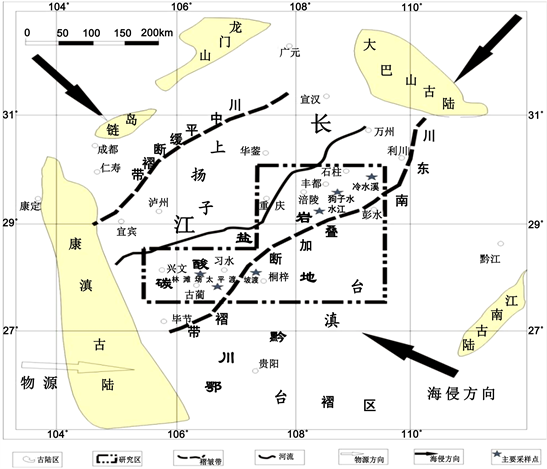Figure 1. Overview of the study area

3. TOC测井响应特征

4. 交会图法判别分析

4.1. 交会图法原理

4.2. 交会图法优选测井参数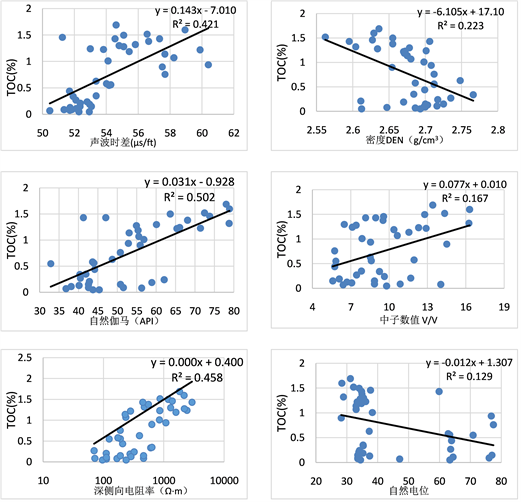Figure 2. The preferred logging curve of the intersection chart method

5. 交叉验证优选支持向量机参数

SVM判别方法的准确性直接取决于核心函数参数γ和惩罚因子C的选择。核心函数γ能够有效地将高维度的映射转变成方便的点乘，核心函数还可以定义特征空间。选择恰当的核心函数，将研究数据映射到适当的样本空间中，才能将支持向量机性能提升到高值。惩罚因子C是用来权衡损失和分类间隔的权重，因子越大从而损失越大。假如不断提高因子数值，在一定程度上会实现将样本点完全正确的分类，但又会导致过度拟合使得泛化能力不足  。

$mse=\frac{1}{N}{\sum }_{T=1}^{N}{\left({y}_{c}-y\right)}^{2}$

R2是拟合优度，可以衡量回归方程整体的拟合程度，R2最大值为1，R2的值越接近1，说明回归直线对观测值的拟合程度越好，反之，R2的值越接近0，拟合程度越差。

${R}^{2}=\frac{{\sum \left({y}_{c}-\stackrel{¯}{y}\right)}^{2}}{{\sum \left(y-\stackrel{¯}{y}\right)}^{2}}或{R}^{2}=1-\frac{{\sum \left(y-{y}_{c}\right)}^{2}}{{\sum \left(y-\stackrel{¯}{y}\right)}^{2}}$

6. 建立SVM回归预测模型

6.1. 支持向量机与回归预测原理

6.1.1. 线性可分样本集

${w}^{*}\cdot x+{b}^{*}\text{=}0$ (1)

$f\left(x\right)=sign\left({w}^{*}\cdot x+{b}^{*}\right)或f\left(x\right)=sign\left({\sum }_{i=1}^{N}{a}_{i}^{*}{y}_{i}〈{x}_{i},x〉+{b}^{*}\right)$ (2)

${\mathrm{min}}_{w,b}=\frac{1}{2}{‖w‖}^{2}$ (3)

$s.t.\text{}{y}_{i}\left(w\cdot {x}_{i}+b\right)-1\ge 0,i=1,\cdots ,N$

6.1.2. 非线性可分样本集

$\underset{\omega ,b,\xi }{\mathrm{min}}\frac{1}{2}{‖\omega ‖}^{2}+C\underset{i=1}{\overset{N}{\sum }}{\xi }_{i}$ (4)

$s.t.\text{}{y}_{i}\left({\omega }_{i}+b\right)\ge 1-{\xi }_{i};i=1,2,\cdots ,N\text{}{\xi }_{i}\ge 0;i=1,2,\cdots ,N$

$f\left(x\right)=sign\left(\underset{i-1}{\overset{N}{\sum }}{a}_{i}^{*}{y}_{i}k\left({x}_{i},x\right)+{b}^{*}\right)$ (5)

6.2. 支持向量机模型构建

$\begin{array}{l}f\left(x\right)=\omega \cdot \varphi \left(x\right)+b\\ \text{}=\underset{i=1}{\overset{n}{\sum }}\left({a}_{i}^{*}-{a}_{i}\right)K\left({x}_{i}\cdot x\right)+{b}^{*}\end{array}$ (6)

$\omega$ ——权数；

$\varphi \left(x\right)$ ——非线性映射函数集合；

b——阈值；

${a}_{i}^{*}$${a}_{i}$ 、b——可通过某点数值计算得到的模型参数 $K\left({x}_{i}\cdot x\right)$ 为核函数且满足下式：

$K\left({x}_{i}\cdot x\right)=\mathrm{exp}\left(1-\frac{{|x-{x}_{i}|}^{2}}{{\sigma }^{2}}\right)$ (7)

6.3. 训练集和测试集回归预测结果精度图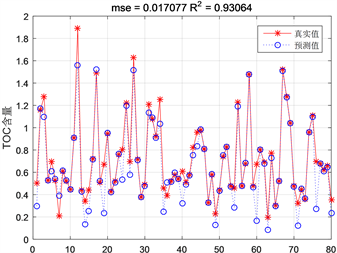Figure 3. Comparison graph of training set results of SVM prediction model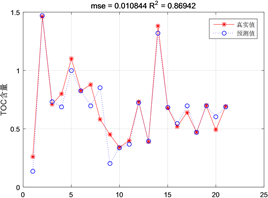Figure 4. Comparison diagram of test set results of SVM prediction model

7. 应用实例分析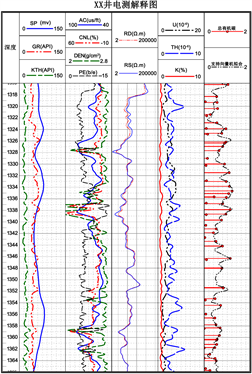Figure 5. Comparison of results of organic carbon content in well XX

8. 结论

1) 训练集与测试集的测试结果相差不大，且拟合度普遍较高，平均平方误差小，但对于含量偏低的数据测试不佳，测试模型还有所欠缺。

2) 经过实验测试，利用支持向量机拟合一定范围内的TOC值有较高的准确率，支持向量机不失为探测TOC值的新方法。

  曲彦胜, 钟宁宁, 刘岩, 等. 烃源岩有机质丰度的测井计算方法及影响因素探讨[J]. 岩性油气藏, 2011, 23(2): 80-84+99.  许晓宏, 黄海平, 卢松年. 测井资料与烃源岩有机碳含量的定量关系研究[J]. 江汉石油学院学报, 1998(3): 11-15.  朱志军, 陈洪德. 川东南地区早志留世晚期沉积特征及沉积模式分析[J]. 中国地质, 2012, 39(1): 64-76.  杨涛涛, 范国章, 吕福亮, 等. 烃源岩测井响应特征及识别评价方法[J]. 天然气地球科学, 2013, 24(2): 414-422.  魏文文, 周大宇. 优质烃源岩识别标志与控制因素[J]. 内蒙古石油化工, 2010, 36(17): 10-11.  周国清. 应用MATLAB 软件处理曲线拟合[J]. 重庆职业技术学院学报, 2003, 2(1): 38-39.  高艳芳, 陈实, 冯斌. 交叉验证在离散数据网格化时的应用[J]. 物探化探计算技术, 2012, 34(5): 619-621.  王怀亮. 交叉验证在数据建模模型选择中的应用[J]. 商业经济, 2011(10): 20-21.  彭涛, 张翔. 支持向量机及其在石油勘探开发中的应用综述[J]. 勘探地球物理进展, 2007, 30(2): 91-95.  陈科贵, 吴刘磊, 陈愿愿, 等. 基于支持向量机的川中杂卤石分类识别研究[J]. 地球科学进展, 2016, 31(10): 1041-1046.  陈金凤. 支持向量机回归算法的研究与应用[D]: [硕士学位论文]. 无锡: 江南大学, 2008.  周卫国. 基于支持向量机的大坝基础注浆量预测模型研究[J]. 水利技术监督, 2018(6): 157-160.